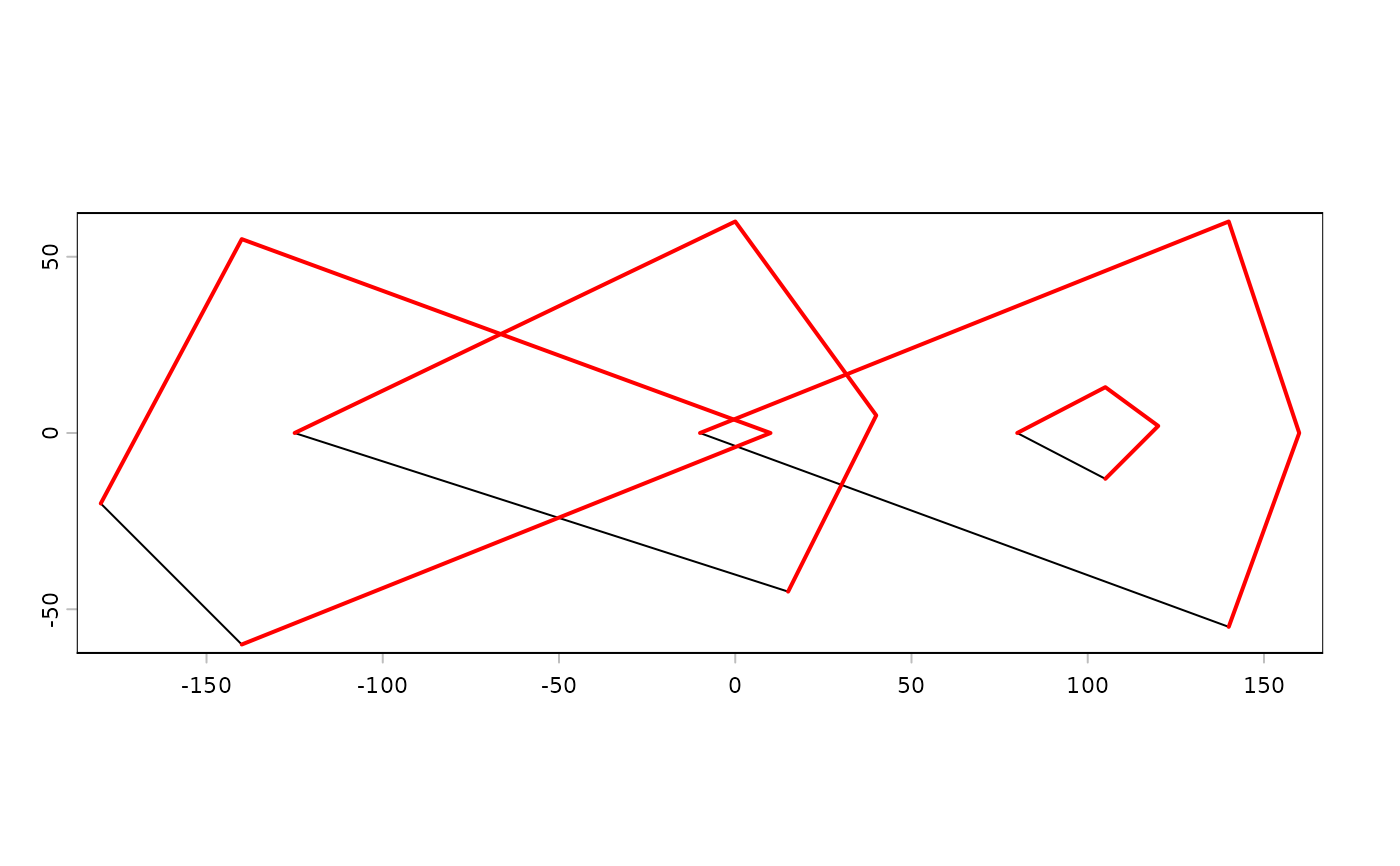Methods to create a SpatVector from a filename or other R object.

A filename can be for a shapefile or any spatial file format.

You can use a data.frame to make a SpatVector of points; or a "geom" matrix to make a SpatVector of any supported geometry (see examples and geom).

You can supply a list of SpatVectors to append them into a single SpatVector.

SpatVectors can also be created from "Well Known Text", and from spatial vector data objects defined in the sf or sp packages.

## Usage

# S4 method for character
vect(x, layer="", query="", extent=NULL, filter=NULL,
crs="", proxy=FALSE, what="")

# S4 method for matrix
vect(x, type="points", atts=NULL, crs="")

# S4 method for data.frame
vect(x, geom=c("lon", "lat"), crs="", keepgeom=FALSE)

# S4 method for list
vect(x, type="points", crs="")

# S4 method for SpatExtent
vect(x, crs="")

# S4 method for SpatVectorCollection
vect(x)

# S4 method for sf
vect(x)

## Arguments

x

character. A filename; or a "Well Known Text" string; SpatExtent, data.frame (to make a SpatVector of points); a "geom" matrix to make a SpatVector of any supported geometry (see examples and geom); a spatial vector data object defined in the sf or sp packages; or a list with matrices with coordinates

layer

character. layer name to select a layer from a file (database) with multiple layers

query

character. An query to subset the dataset in the OGR-SQL dialect

extent

Spat* object. The extent of the object is used as a spatial filter to select the geometries to read. Ignored if filter is not NULL

filter

SpatVector. Used as a spatial filter to select geometries to read (the convex hull is used for lines or points). It is guaranteed that all features that overlap with the extent of filter will be returned. It can happen that additional geometries are returned

type

character. Geometry type. Must be "points", "lines", or "polygons"

atts

data.frame with the attributes. The number of rows must match the number of geometrical elements

crs

character. The coordinate reference system in one of the following formats: WKT/WKT2, <authority>:<code>, or PROJ-string notation (see crs). See crs

proxy

logical. If TRUE a SpatVectorProxy is returned

what

character indicating what to read. Either "" for geometries and attributes, or "geoms" to only read the geometries, "attributes" to only read the attributes (that are returned as a data.frame)

geom

character. The field name(s) with the geometry data. Either two names for x and y coordinates of points, or a single name for a single column with WKT geometries)

keepgeom

logical. If TRUE the geom variable(s) is (are) also included in the attributes

geom

SpatVector

## Examples

### SpatVector from file
f <- system.file("ex/lux.shp", package="terra")
f
#>  "/Users/runner/work/_temp/Library/terra/ex/lux.shp"
v <- vect(f)
v
#>  class       : SpatVector
#>  geometry    : polygons
#>  dimensions  : 12, 6  (geometries, attributes)
#>  extent      : 5.74414, 6.528252, 49.44781, 50.18162  (xmin, xmax, ymin, ymax)
#>  source      : lux.shp
#>  coord. ref. : lon/lat WGS 84 (EPSG:4326)
#>  names       :  ID_1   NAME_1  ID_2   NAME_2  AREA   POP
#>  type        : <num>    <chr> <num>    <chr> <num> <int>
#>  values      :     1 Diekirch     1 Clervaux   312 18081
#>                    1 Diekirch     2 Diekirch   218 32543
#>                    1 Diekirch     3  Redange   259 18664

## subsetting (large) files
## with attribute query
v <- vect(f, query="SELECT NAME_1, NAME_2, ID_2 FROM lux WHERE ID_2 < 4")

## with an extent
e <- ext(5.9, 6.3, 49.9, 50)
v <- vect(f, extent=e)

## with polygons
p <- as.polygons(e)
v <- vect(f, filter=p)

### SpatVector from a geom matrix
x1 <- rbind(c(-180,-20), c(-140,55), c(10, 0), c(-140,-60))
x2 <- rbind(c(-10,0), c(140,60), c(160,0), c(140,-55))
x3 <- rbind(c(-125,0), c(0,60), c(40,5), c(15,-45))
hole <- rbind(c(80,0), c(105,13), c(120,2), c(105,-13))
z <- rbind(cbind(object=1, part=1, x1, hole=0), cbind(object=2, part=1, x3, hole=0),
cbind(object=3, part=1, x2, hole=0), cbind(object=3, part=1, hole, hole=1))
colnames(z)[3:4] <- c('x', 'y')

p <- vect(z, "polygons")
p
#>  class       : SpatVector
#>  geometry    : polygons
#>  dimensions  : 3, 0  (geometries, attributes)
#>  extent      : -180, 160, -60, 60  (xmin, xmax, ymin, ymax)
#>  coord. ref. :

z[z[, "hole"]==1, "object"] <- 4
lns <- vect(z[,1:4], "lines")
plot(p)
lines(lns, col="red", lwd=2)### from wkt
v <- vect("POLYGON ((0 -5, 10 0, 10 -10, 0 -5))")

wkt <- c("MULTIPOLYGON ( ((40 40, 20 45, 45 30, 40 40)),
((20 35, 10 30, 10 10, 30 5, 45 20, 20 35),(30 20, 20 15, 20 25, 30 20)))",
"POLYGON ((0 -5, 10 0, 10 -10, 0 -5))")
w <- vect(wkt)

# combine two SpatVectors
vw <- rbind(w, v)

d <- data.frame(id=1:2, name=c("a", "b"))
values(w) <- d

# add data.frame on creation, here from a geom matrix
g <- geom(w)
d <- data.frame(id=1:2, name=c("a", "b"))
m <- vect(g, "polygons", atts=d, crs="+proj=longlat +datum=WGS84")

### SpatVector from a data.frame
d$wkt <- wkt x <- vect(d, geom="wkt") d$wkt <- NULL
d$lon <- c(0,10) d$lat <- c(0,10)
x <- vect(d, geom=c("lon", "lat"))

# SpatVector to sf
#sf::st_as_sf(x)